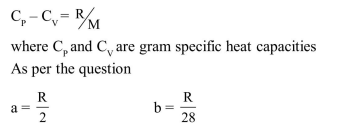Deepak Scored 45->99%ile with Bounce Back Crack Course. You can do it too!

# Solve this following

Question:

$\mathrm{C}_{\mathrm{p}}$ and $\mathrm{C}_{\mathrm{v}}$ are specific heats at constant pressure and constant volume respectively. It is observed that $C_{p}-C_{v}=a$ for hydrogen gas $C_{p}-C_{v}=b$ for nitrogen gas The correct relation between a and $b$ is :

1. $a=14 b$

2. $a=28 b$

3. $a=\frac{1}{14} b$

4. $a=b$

Correct Option: 1

Solution: